• 1、“右击MATLAB”图标，打开...3、复制这个文件，放到U盘、云盘、邮箱之类的，目的是拷贝到另一台PC上。 4、将文件在解压到另一台D盘。（最好不要C盘，其它都行） 5、MATLAB安装目录中，大写的MATLAB.ex...
1、“右击MATLAB”图标，打开文件所在目录。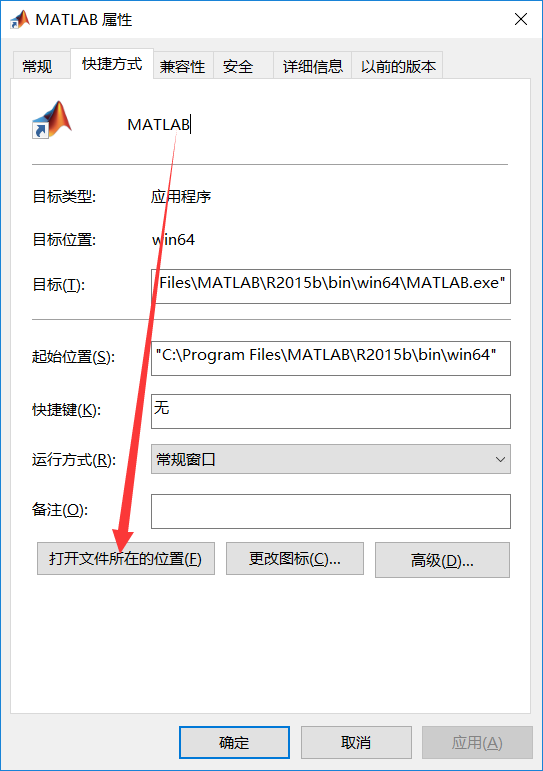2、后退，然后复制MATLAB里面的那个文件夹（我的是R2015b）（MATLAB这个文件夹要不要都可以）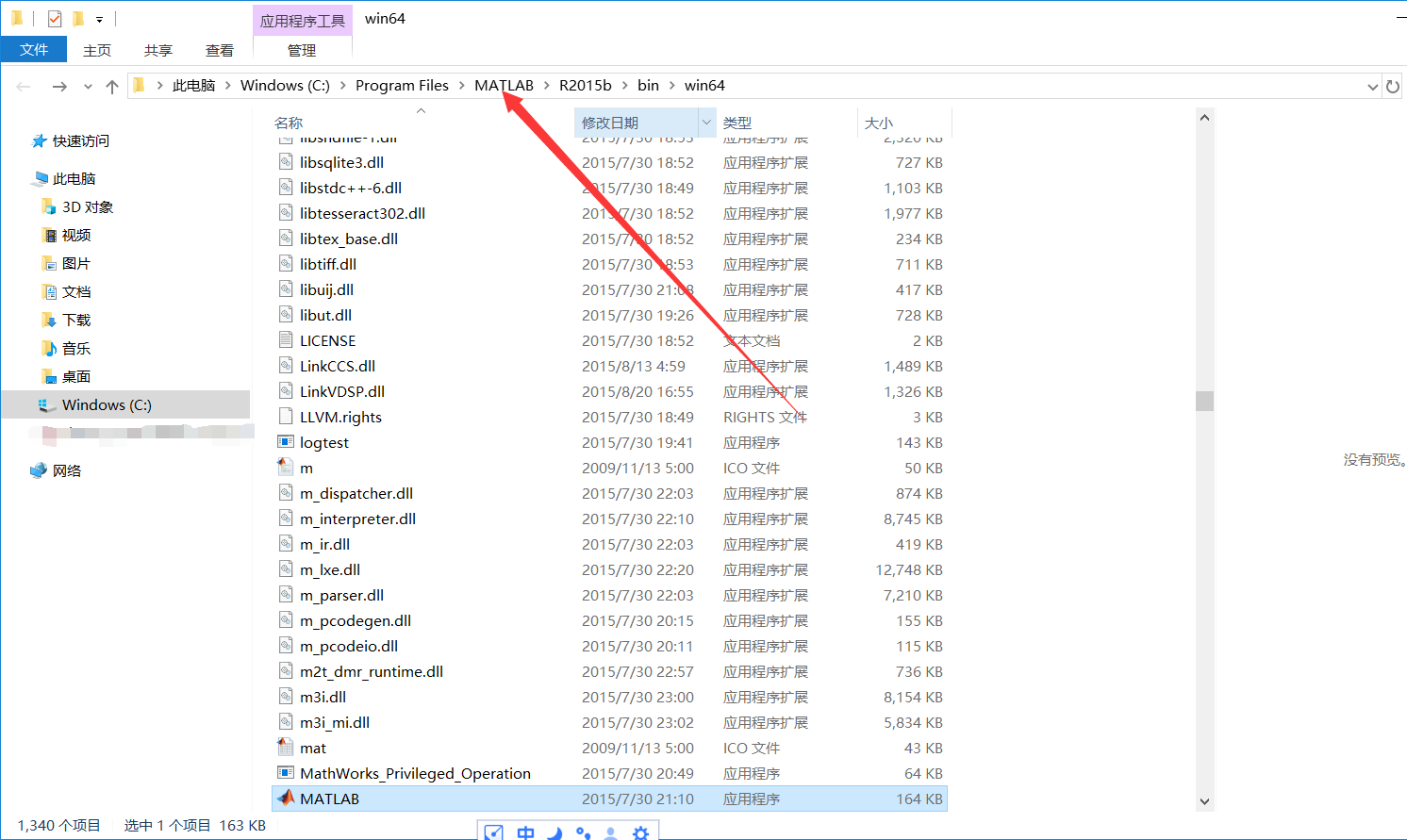3、复制这个文件，放到U盘、云盘、邮箱之类的，目的是拷贝到另一台PC上。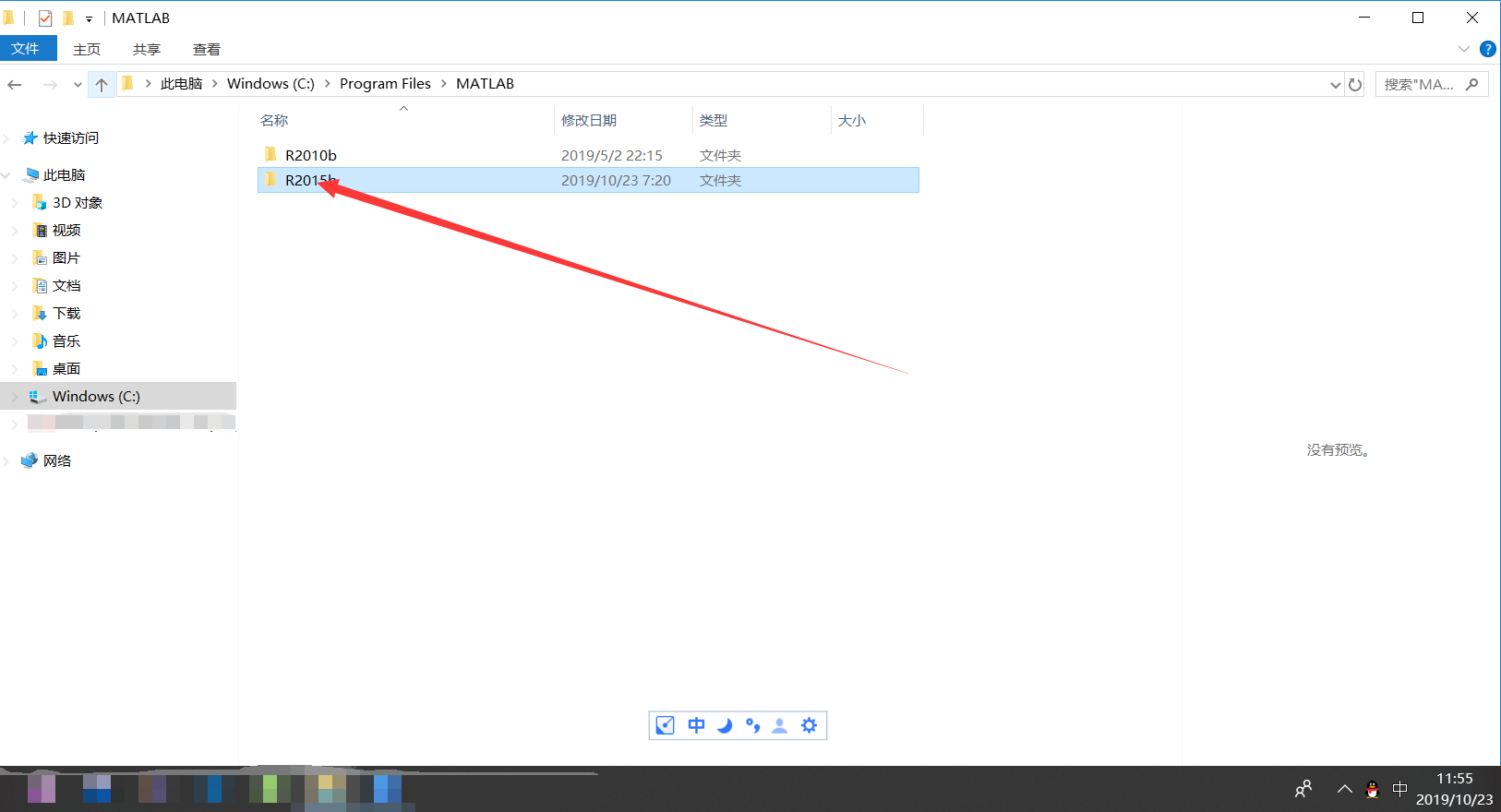4、将文件在解压到另一台D盘。（最好不要C盘，其它都行）

5、 MATLAB安装目录中，大写的MATLAB.exe(大部分WIN10隐藏后缀)为MATLAB的启动程序,双击即可启动MATLAB。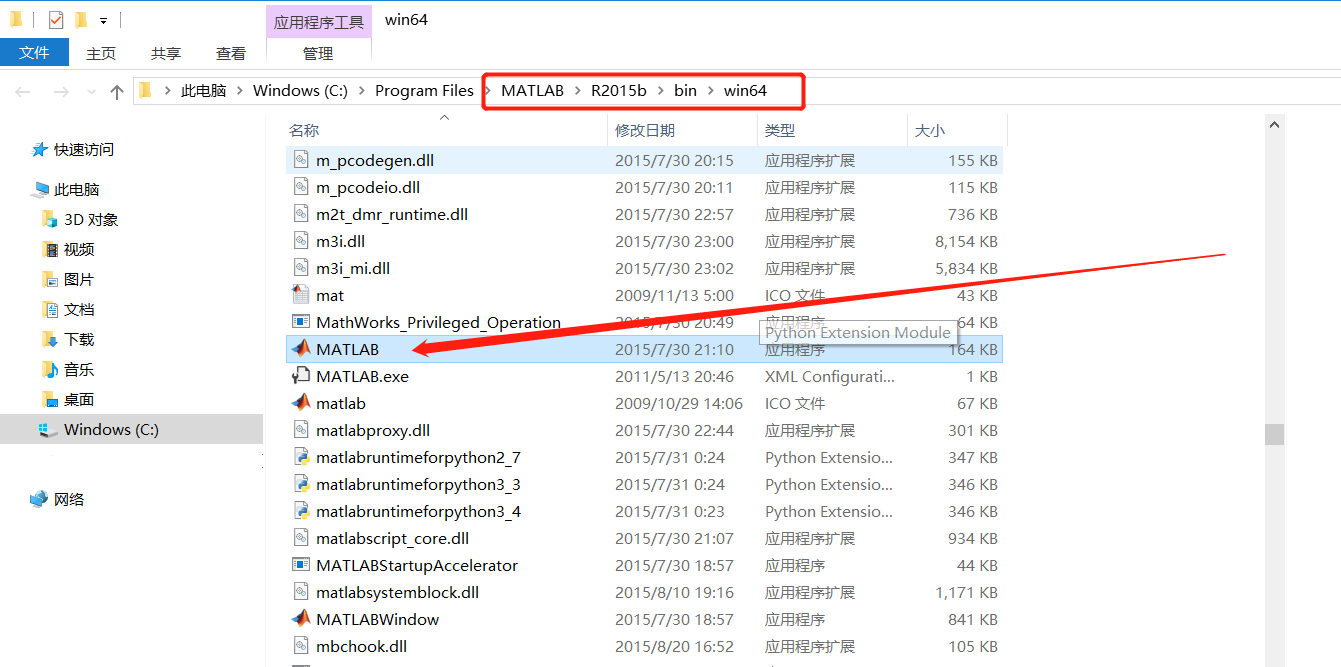6、也可以在桌面设置快捷方式。

“右击”——>“新建”——>“快捷方式”。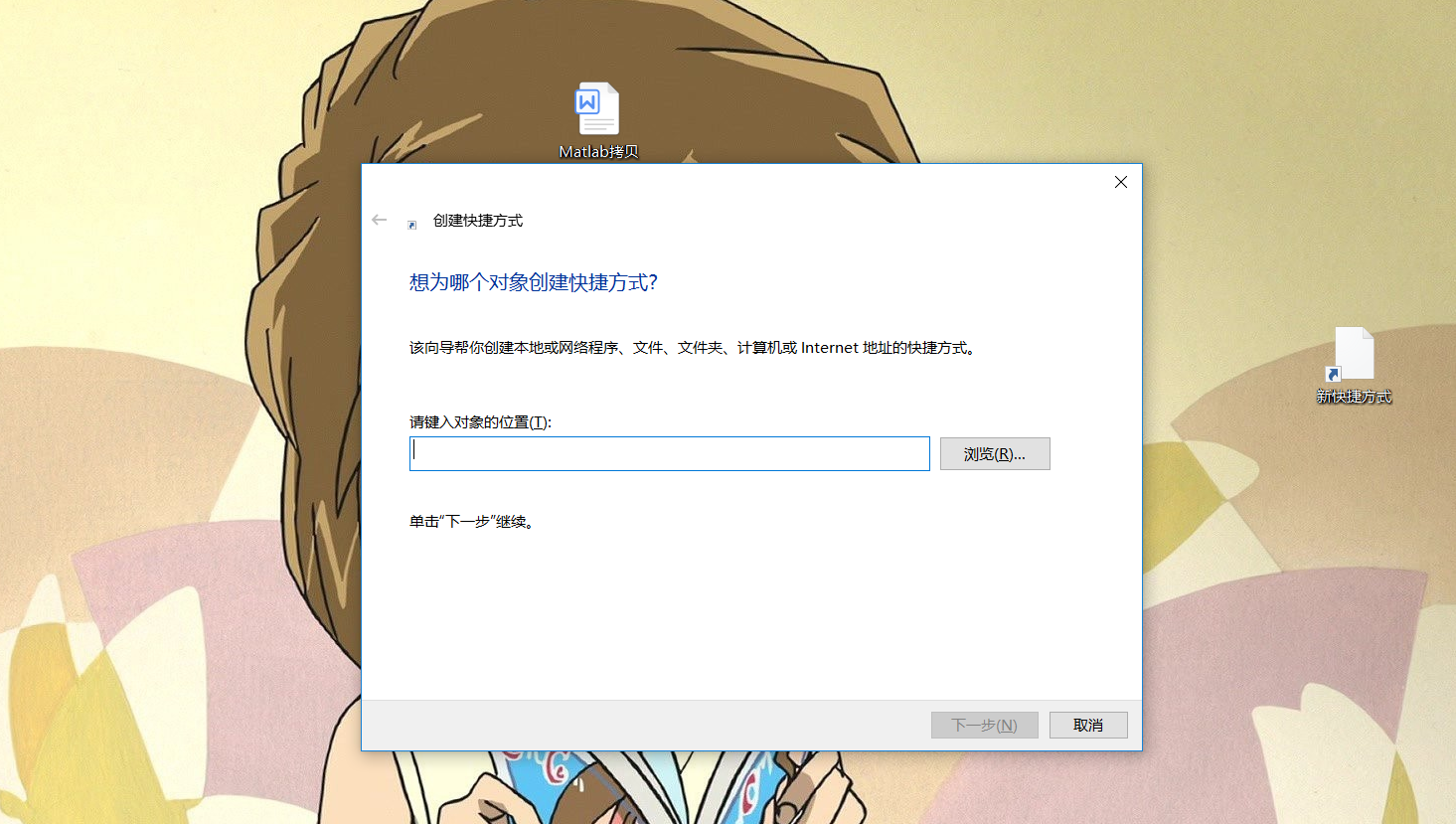点击“浏览”，找到【5】中的那个启动应用程序，选中。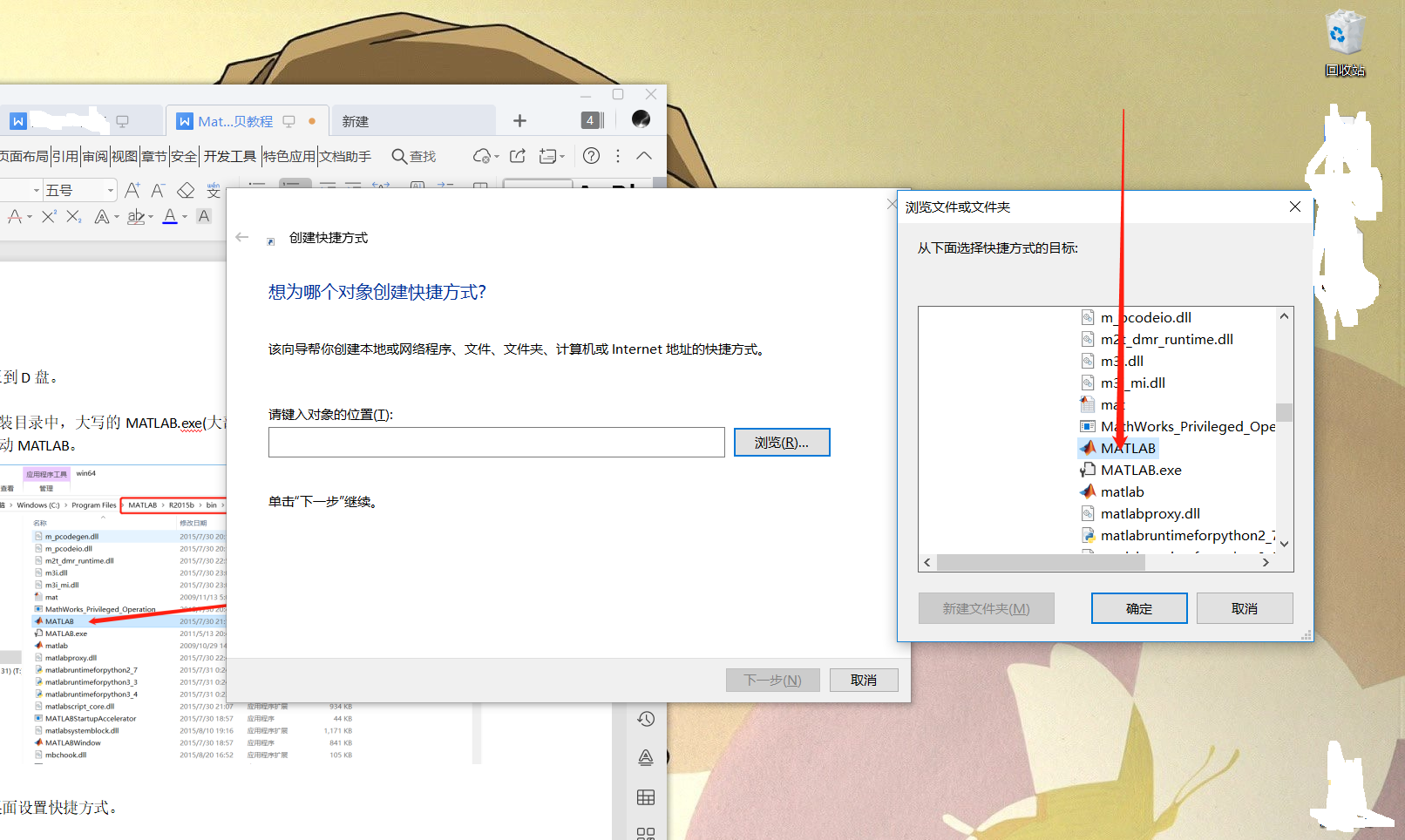“下一步”——>“完成”。感叹一下！
Matlab越来越大了。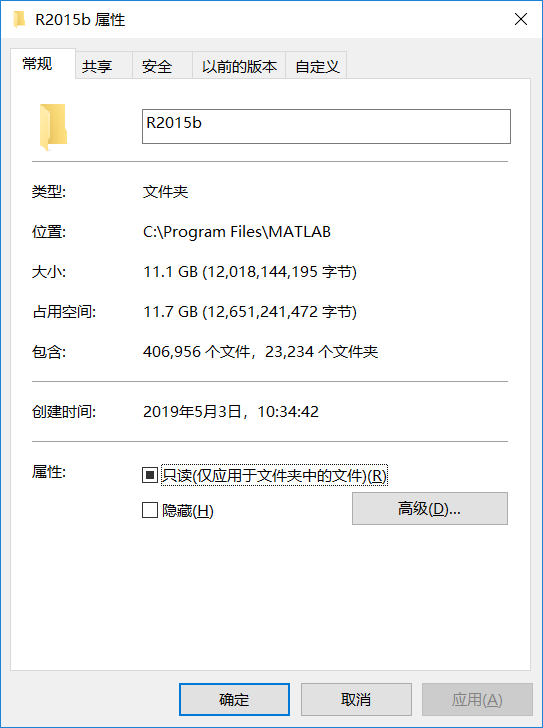展开全文• sourcePath = '.\Square_train'; targetPath = '.\Square_test'; fileList = dir(sourcePath); for k = 3 :5: length(fileList) movefile([sourcePath,'\',fileList(k).name],targetPath);...
sourcePath = '.\Square_train';   targetPath = '.\Square_test';
fileList = dir(sourcePath);      for k = 3 :5: length(fileList)       movefile([sourcePath,'\',fileList(k).name],targetPath); end
%copyfile([sourcePath,'\',fileList(k).name],targetPath)  
展开全文• clear clc cd('C:\Documents and Settings\...% 此时test文件夹中有:文件夹1, 文件夹2， 文件1.txt, 文件2.txt movefile('1.txt', '11.txt'); % 把1.txt剪切成11.txt(1.txt不存在了)，实际上相当于...
clear
clc

%  此时test文件夹中有:文件夹1, 文件夹2， 文件1.txt, 文件2.txt

movefile('1.txt', '11.txt');  % 把1.txt剪切成11.txt(1.txt不存在了)，实际上相当于改名
copyfile('2.txt', '22.txt');  % 把2.txt复制成22.txt(2.txt依然存在)

movefile('11.txt', '1');      % 把11.txt剪切到文件夹1中
copyfile('22.txt', '2');      % 把22.txt复制到文件夹2中
展开全文• ## matlab中文件的复制

千次阅读 2015-09-18 11:21:37
下面这个程序完成的功能就是根据聚类之后的结果，把相对应的波形csd文件拷贝到相应的类别中。clc; clear; NODE='OUTPUT1'; path_fault='G:\wuproject\wu123\CSDF_Files\fault\';//源文件地址 path_cluster='G:\...
下面这个程序完成的功能就是根据聚类之后的结果，把相对应的波形csd文件拷贝到相应的类别中。
clc;
clear;
NODE='OUTPUT1';
path_fault='G:\wuproject\wu123\CSDF_Files\fault\';//源文件地址
path_cluster='G:\matlab_work\二分K均值聚类bywu注释\';//目的地址
if path_fault(end)~='\'
path_fault=[path_fault,'\'];
end
if path_cluster(end)~='\'
path_cluster=[path_cluster,'\'];
end
[cluster_list_row  cluster_list_col]=size(cluster_list);
%将cluster_list中的每个类中对应的fault波形保存在指定的路径下
DIRS=dir([path_fault,'*.csd']);  %扩展名
n=length(DIRS);
for k=1:cluster_list_col
for i_csd_num=1:n %把每个csd文件的序号提取出来，看每个类中是否包含此序号，若包含有，则进行拷贝
if  find(cluster_list{1,k}{1,2}==i_csd_num)>0
path_dist=[path_cluster,NODE,'\cluster-',num2str(k),'\'];
path_source=[path_fault,DIRS( i_csd_num).name];
if  ~isdir(path_dist)
mkdir(path_dist);
end
copyfile(path_source,path_dist);
end
end
end
上面是自己在项目中遇到的一个实际的例子，关于文件的复制。下面就将matlab文件的复制这个功能从项目中提取出来，并举一个例子来进行讲解。  文件的复制的知识点就是
copyfile(path_source,path_dist);
例子代码如下
function copy
% 从father目录中复制指定类型的文件到目录s中
father='H:\前期测试\3\'; %指定类型的文件所在的目录
s='H:\前期测试\3.3\'; %复制文件的目标目录
subDir=dir(father); %求目录的子目录
len = length(subDir); %求子目录的长度
disp('begin copy files..');
for i=3:len
imgNames = dir(strcat(father,subDir(i).name,'\','*.JPEG'));
a=[s,subDir(i).name,'\'];
mkdir([s,subDir(i).name])
for j=1:20 %复制的文件个数
copyfile([father,subDir(i).name,'\',imgNames(j).name],a);
end
end
disp('end');
end
展开全文• %% move_img % Summary of example objective clear;clc; SOURCE_PATH_t = 'D:\voc\2007\JPEGImages\';%源文件目录 DST_PATH_t = 'D:\voc\2007\xin_2007\'...%目的文件目录 for i=1:9963 filename=['D:\v
• 环境 ...Ubuntu 14.04Matlab 2014b ...将matlab代码从Windows中复制到Linux中（或从Linux复制到Windows）时，matlab代码中的中文注释部分变成了乱码。 原因 Windows和Linux中的默认编码不同，Wi
• 代码 srcpath= 'F:\HeartMultiAtlas\MM-WHS2017\OrCa2\ct_atlas\'; dstpath=' F:\ResData\mask\ '; filename=' * LV. nii' ; fdt = dir( [srcpath filename] ); for ...% 拷贝文件 end end
• MATLAB，文件夹或文件的，创建、复制、移动、删除、重命名等等文件夹操作
• ## matlab 画图

千次阅读 2019-09-08 14:47:20
本文讲如何使用 matlab 画图。 本文包括：折线图的 x轴和y轴、标题、图例 柱状图填充图案
• 文件批量复制、剪切和修改文件名批量复制新的改变功能快捷键合理的创建标题，有助于目录的生成如何改变文本的样式插入链接与图片如何插入一段漂亮的代码片生成一个适合你的列表创建一个表格设定内容居中、居左、居右...批量复制 批量修改文件名
• sourcePath = ' '; %原来路径 targetPath = ' '; %目标路径 fileList = dir(sourcePath); movefile([sourcePath,'\...%copyfile([sourcePath,'\',fileList(k).name],targetPath)%复制文件，原来路径还有文件 ...
• copyfile('source','destination')；%复制文件 delete('fileName');%删除文件 movefile('source','destination');%移动文件 　 转载于:https://www.cnblogs.com/hsy1941/p/7191168.html...
• 如何将一个波形文件下载到安捷伦信号发生器中，并在信号发生器中调用波形文件的具体操作流程
• ## matlab生成EXE文件

千次阅读 2018-06-11 08:46:14
原文通常编 写的matlab程序只能在装有matlab的机器上执行，但是没有安装matlab的怎么办呢？这个可以使用matlab的自带工具-Deploy Tool。 Matlab的Deploy Tools 可以方便的将matlab工程编译成 所支持的类型，例如 ...
• Matlab实现文件读写的几种方法 转载 2016年04月12日 15:43:13 标签：
• 如果想将A路径下的n个文件夹移动到将B路径下的n个文件夹下 path='D:\traingabor\train\'; %原图片路径 path1='D:\traingabor\train1\'; %待存放图片路径 for i=1:3600 %3600个文件夹  hz=[path,num2str(i),'\']...
• m文件代码装饰 Matlab GUI以适合从定位显微镜（STORM / PALM）数据获得的数据的概率分布函数（pdf）。 将数据分解为通过校准函数f 1的迭代COvolution所获得的函数f n的加权总和。 f 1的功能形式及其参数必须通过独立...
• Matlab.m文件生成.exe文件之旅 Matlab中.m文件生成.exe文件之旅 作为算法工程师，有时需要测试人员帮忙测试算法模型，故需要向其发布一个算法工具，也是就是.exe文件。由于近期有利用MATLAB进行相关的工作，故在此...
• matlab中有很多写好的库函数可以方便使用，想使用matlab自己的数据文件.mat文件也需要引用matlab的相关库函数。 具体有两种用法： 1、在matlab中写好相关函数，发布为dll供Qt使用 2、直接在Qt中使用matlab库函数 ...Qt mat
• matlab中将mat数据文件转成excel文件 最近处理光伏数据全是m文件，暂时还没找到python里可以简单调用的方法，用matlab处理了一下，可以转换成Excel或者csv文件，非常方便！ 我们对Markdown编辑器进行了一些功能拓展...python excel
• 有时候数据处理需要载入整个文件夹，本教程提出一种利用路径选择对话框uigetdir 和文件复制命令copyfile 实现的MATLAB复制载入整个数据文件到指定路径下的简单实现代码编程。 方法/步骤 1 启动MATLAB后在...
• 从剪贴板中检索数据。 剪贴板数据可能有多种类型，并封装在自描述结构中。... 在 Windows XP、Mac OS... 新增功能：您可以从剪贴板粘贴文件句柄（带路径）。 您可以使用剪贴板粘贴功能，也可以使用新的便利功能 clipfile。
• 使用matlab时的注意事项，比如不能按CTRL+Q 语句后面加分号，不是交互式，不加分号，会同时打印结果，即交互式。 字符串使用单引号才可以。 变量赋值不需要提前声明，随时使用。 clear清除变量，clc清除屏幕。 ...入门 新手教程 显示
• matlab 生成可执行exe文件的方法虐了好久，终于找到一个有效的方法，分享给大家。  运行m文件，在命令窗口输入mcc -m 文件名.m，回车，在当前文件夹下新生成四个文件（有一个是文件名.exe），将四个文件放到一个...gui 软件
• 首先，P文件为了保护知识产权...因为当你第一次执行M文件时，Matlab需要将其解析（parse）一次（第一次执行后的已解析内容会放入内存作第二次执行时使用，即第二次执行时无需再解析），这无形中增加了执行时间。所以图像处理
• VS与matlab混编之VS调用matlab文件 很长一段时间以来，很多算法工程师会基于matlab进行算法原型的开发，然后移植到C++等语言进行实时验证。但移植的过程，本身是浪费时间的，需要两个语言的转换，甚至不同的工程师...c++...

# matlab拷贝文件matlab 订阅